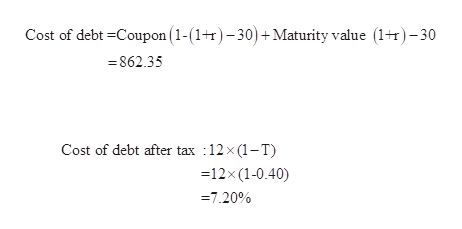# A firm can issue a 15-year, \$1,000 par value bond with a 10% coupon rate, paying interest semiannually, at a price of \$862.35. Their marginal tax rate is 40%. A dividend of \$1.25 was just paid on the firm's  common stock, and the firm's estimated growth rate is 8%. It can issue new common stock at \$25.00 with a 20% floatation cost. The Risk-Free Return is 6%, the Market Return is 12%, and the firm's  beta is 1.2.A) Calculate the firm's Cost of Debt, after Tax.B) Calculate the firm's Cost of Retained Earnings using the CAPM.C) Calculate the firm's Cost of new Common Stock.D) A firm has the following component costs of capital:       Cost of Debt (after-tax):     10.5%       Cost of Retained Earnings: 15.0%    It's target capital structure is:        Debt     40%        Retained Earnings:     60%  Estimate the firm's Weighted Average Cost of Capital (WACC)

Question
10 views

A firm can issue a 15-year, \$1,000 par value bond with a 10% coupon rate, paying interest semiannually, at a price of \$862.35. Their marginal tax rate is 40%. A dividend of \$1.25 was just paid on the firm's  common stock, and the firm's estimated growth rate is 8%. It can issue new common stock at \$25.00 with a 20% floatation cost. The Risk-Free Return is 6%, the Market Return is 12%, and the firm's  beta is 1.2.

A) Calculate the firm's Cost of Debt, after Tax.

B) Calculate the firm's Cost of Retained Earnings using the CAPM.

C) Calculate the firm's Cost of new Common Stock.

D) A firm has the following component costs of capital:

Cost of Debt (after-tax):     10.5%

Cost of Retained Earnings: 15.0%

It's target capital structure is:

Debt     40%

Retained Earnings:     60%

Estimate the firm's Weighted Average Cost of Capital (WACC)

check_circle

Step 1

A)

Computation of firm’s cost of debt after tax:

From the above equation we find the r. If we substitute 12% which means (0.06 being semi-annually equivalent) above equation is satisfied .which means the cost of debt 12% before applying tax rate.

Hence, the cost of debt after tax is 7.20%.help_outlineImage TranscriptioncloseCost of debt -Coupon (1-(1+r)-30)+Maturity value (1+r) -30 862.35 Cost of debt after tax 12x (1-T) 12x (1-0.40) 7.20% fullscreen
Step 2

B)

Computation of cost of retained earnings using CAPM:

Hence, the retained earnings is 13.20%.

...

### Want to see the full answer?

See Solution

#### Want to see this answer and more?

Solutions are written by subject experts who are available 24/7. Questions are typically answered within 1 hour.*

See Solution
*Response times may vary by subject and question.
Tagged in